# Texas Go Math Grade 8 Lesson 7.3 Answer Key Angle-Angle Similarity

Refer to our Texas Go Math Grade 8 Answer Key Pdf to score good marks in the exams. Test yourself by practicing the problems from Texas Go Math Grade 8 Lesson 7.3 Answer Key Angle-Angle Similarity.

## Texas Go Math Grade 8 Lesson 7.3 Answer Key Angle-Angle Similarity

Essential Question
How can you determine when two triangles are similar?

Explore Activity 1

Discovering Angle-Angle Similarity
Similar figures have the same shape but may have different sizes. Two triangles are similar if their corresponding angles are congruent and the lengths of their corresponding sides are proportional.

A. Use your protractor and a straightedge to draw a triangle. Make one angle measure 45° and another angle measure 60°.
B. Compare your triangle to those drawn by your
classmates. How are the triangles the same?

How are they different?

C. Use the Triangle Sum Theorem to find the measure of the third angle of your triangle.

Reflect

Question 1.
If two angles in one triangle are congruent to two angles in another triangle, what do you know about the third pair of angles?
If two angles in one triangle are congruent to two angles in another triangle, then we can call them corresponding
angles of the two triangles since they are congruent

Also, if two pairs of corresponding angles of triangle are congruent then the triangles are similar

By the description of the angles of the two triangles, we can say that the third pair of the angles are also congruent.

Question 2.
Make a Conjecture Are two pairs of congruent angles enough information to conclude that two triangles are similar? Explain.
If two pairs of corresponding angles in a pair of triangles are congruent, it means that the third pair must also be equal since the total sum of interior angles of a triangle is 180°. Therefore, we can conclude that the triangles are similar.

Question 3.
Explain whether the triangles are similar.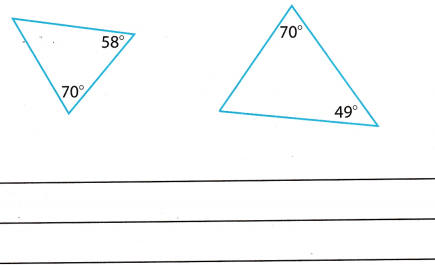180° = 70° + 58° + N° First find what angle N equals
180° = 128° + N°
52° = N°
180° = 70° + 49° + Q° Then find what angle Q equals
180° = 119° + Q°
61° = Q°
Since one angle from these triangles are the same then these are not similar triangles.

Example 2.
While playing tennis, Matt is 12 meters from the net, which is 0.9 meter high. He needs to hit the ball so that it just clears the net and lands 6 meters beyond the base of the net. At what height should Matt hit the tennis ball?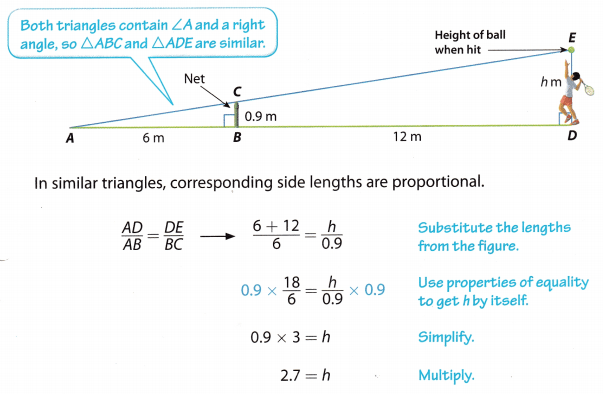Matt should hit the ball at a height of 2.7 meters.

Reflect

Question 4.
What If? Suppose you set up a proportion so that each ratio compares parts of one triangle, as shown below.Show that this proportion leads to the same value for h as in Example 2.
We are watching triangles ∆ABC and ∆ABD. Both triangles contain ∠A and a right angle, so ∆ABC and ∆ADE are similar. Lets set up a proportion: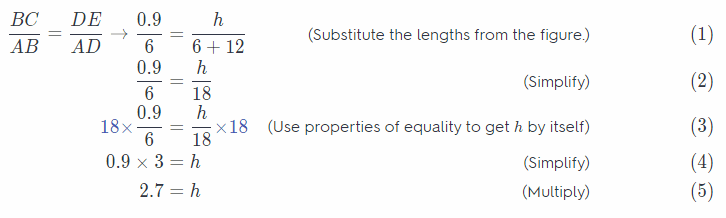This proportion leads to the same value for h as in ExampLe 2

Question 5.
Rosie is building a wheelchair ramp that is 24 feet long and 2 feet high. She needs to install a vertical support piece 8 feet from the end of the ramp. What is the length of the support piece in inches?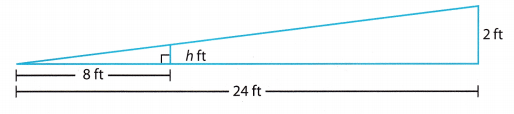Triangles formed from wheelchair ramp, supported piece, and distances between are forming similar triangles.
Lets set up a proportion using known lengths
$$\frac{8}{24}$$ = $$\frac{h}{2}$$ (Substitute the lengths from the figure.) (1)
2 × $$\frac{8}{24}$$ = $$\frac{h}{2}$$ × 2 (Use properties of equality to get h by itself) (2)
$$\frac{2}{3}$$ = h (Simplify) (3)
h = $$\frac{2}{3}$$ft = $$\frac{2}{3}$$ . 12 inches = 8 inches (4)

h = 8 inches

Question 6.
The lower cable meets the tree at a height of 6 feet and extends out 16 feet from the base of the tree. If the triangles are similar, how tall is the tree?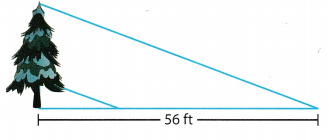Lets set up a proportion using known lengths:
$$\frac{h}{56}$$ = $$\frac{6}{16}$$ (Substitute the lengths from the figure.) (1)
56 × $$\frac{h}{56}$$=$$\frac{6}{16}$$ × 56 (Use properties of equality to get h by itself) (2)
h = 21ft (Simplify) (3)

h = 21ft

Explore Activity 2
Using Similar Triangles to Explain Slope

You can use similar triangles to show that the slope of a line is constant.

A. Draw a line l that is not a horizontal line. Label four points on the line as A, B, C, and D.

B. Draw the rise and run for the slope between points A and B. Label the intersection as point E. Draw the rise and run for the slope between points Cand D. Label the intersection as point F.

C. Write expressions for the slope between A and B and between C and D.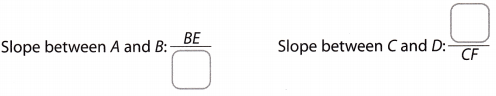D. Extend $$\overleftrightarrow{A E}$$ and $$\overleftrightarrow{C F}$$ across your drawing. $$\overleftrightarrow{A E}$$ and $$\overleftrightarrow{C F}$$ are both horizontal
lines, so they are parallel.
Line l is a _______________ that intersects parallel lines.
E. Complete the following statements:
∠BAE and ________ are corresponding angles and are ___.
∠BEA and ________ are right angles and are ___.
F. By Angle—Angle Similarity, ∠ABE and ___ are similar triangles.
G. Use the fact that the lengths of corresponding sides of similar triangles are proportional to complete the following ratios: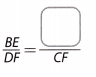H. Recall that you can also write the proportion so that the ratios compare parts of the same triangle:.
I. The proportion you wrote in step H shows that the ratios you wrote in C, are equal. So, the slope of line l is constant.

Reflect

Question 7.
What If? Suppose that you label two other points on line l as G and H. Would the slope between these two points be different than the slope you found in the Explore Activity? Explain.
If we Label two other points on Line as G and H and we follow the steps as in the Explore Activity 2, we will see that
the sLope between these two points will be the same as the one we found before.
First, we draw the rise and run for the slope between points G and H and we label the intersection as point M. We write the expression for the slope between G and H as $$\frac{H M}{G M}$$. We extend GM across our drawing, so it is parallel with the extensions of AE and CF. We find the corresponding and the right angles and we see that the triangles that were formed are similar. Therefore, we write the proportions and we conclude that the slopes are equal.

Question 1.
Explain whether the triangles are similar. Label the angle measures in the figure. (Explore Activity 1 and Example 1)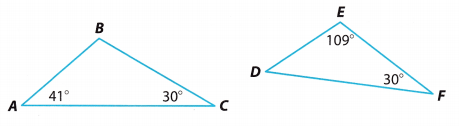∆ABC has angle measures ____________________ and ∆DEF has angle measures _________________. Because ______________ in one triangle are congruent to ________________ in the other triangle, the triangles are ___.
∆ÁBC has angle measures 40°, 30° and 109°
∠B = 180 – A – C = 180 – 41 – 30 = 109°)

and ∆DEF has angle measures 41°, 109°, and 30°
∠D = 180 – E – F = 180 – 109 – 30 = 41°)

Because
2∠s
in the other triangle, the
triangles are similar
40°, 30°, and 109°
41°, 109°. and 30°,
similar

Question 2.
A flagpole casts a shadow 23.5 feet long. At the same time of day, Mrs. Gilbert, who is 5.5 feet tall, casts a shadow that is 7.5 feet long. How tall in feet is the flagpole? Round your answer to the nearest tenth. (Example 2)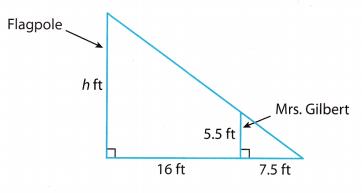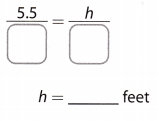In similar triangles, corresponding side lengths are proportional.
$$\frac{5.5}{7.5}$$ = $$\frac{h}{23.5}$$
23.5 . 7.5 . $$\frac{5.5}{7.5}$$ = $$\frac{h}{23.5}$$ . 23.5 . 7.5
23.5 . 5.5 = 7.5 . h
h = $$\frac{129.25}{7.5}$$
Rounding to the nearest tenth:
h = 17.2 feet

Question 3.
Two transversals intersect two parallel lines as shown. Explain whether ∆ABC and ∆DEC are similar. (Example∠BAC and ∠EDC are ____________ since they are _______
∠ABC and ∠DEC are ____________ since they are _______
By ____________, ∆ABC and ∆DEC are ____.
∠BAC and ∠EDC are congruent since they are
alt. interior ∠s
∠ABC and ∠DEC are congruent since they are
alt. interior ∠s
By AA similarity, ∆ABC and ∆DEC are similar.

AA similarity; similar (see explanation)

Essential Question Check-In

Question 4.
How can you determine when two triangles are similar?
If 2 angles of one triangle are congruent to 2 angles of another triangle, the triangles are similar by the Angle-Angle Similarity Postulate

Angle – Angle Similarity Postulate (see explanation)

Use the diagrams for Exercises 5-7.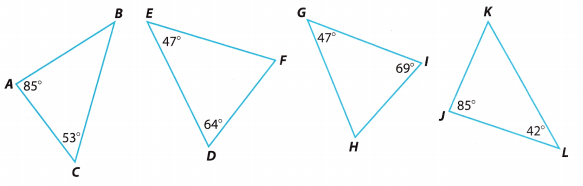Question 5.
Find the missing angle measures in the triangles.
a) From the Triangle Sum Theorem we have:
m∠A + m∠B + m∠C = 180°
We substitute the given angle measures and we solve for m∠B.
85° + m∠B + 53° = 180°
138° + m∠B = 180°
138° – 138° + m∠B = 180° – 138°
m∠B = 42°

b) From the Triangle Sum Theorem we have:
m∠D + m∠E + m∠F = 180°
We substitute the given angle measures and we solve for m∠F.
64° + 47° + m∠F = 180°
111° + m∠F = 180°
111° – 111° + m∠F = 180° – 111°
m∠F = 69°

c) From the Triangle Sum Theorem we have:
m∠G + m∠H + m∠I = 180°
We substitute the given angle measures and we solve for m∠H.
47° + m∠H + 69° = 180°
116° + m∠H = 180°
116° – 116°+ m∠H = 180° – 116°
m∠H = 64°

d) From the Triangle Sum Theorem we have:
m∠J + m∠K + m∠L = 180°
We substitute the given angle measures and we solve for m∠H.
85° + m∠K + 42° = 180°
127° + m∠K = 180°
127° – 127° + m∠K = 180° – 127°
m∠K = 53°

Question 6.
Which triangles are similar?
∆ABC’ and ∆JKL are similar because their corresponding angles are congruent Also, ∆DEF and ∆GHI are similar because their corresponding angles are congruent.

Question 7.
Analyze Relationships Determine which angles are congruent to the angles in A ABC.
∆JKL has angle measures that are the same as those is ∆ÀBC
(∠A ≅ ∠J, ∠B ≅ ∠L, and ∠C ≅ ∠K)
Therefore, they are congruent

∆JKL ≅ ∆ABC

Question 8.
Multistep A tree casts a shadow that is 20 feet long. Frank is 6 feet tall, and while standing next to the tree he casts a shadow that is 4 feet long.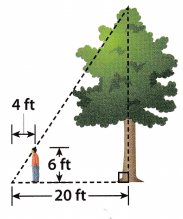a. Flow tall is the tree? _____
In similar triangles, corresponding side lengths are proportional.
$$\frac{20}{4}$$ = $$\frac{h}{6}$$
6 . 4 . $$\frac{20}{4}$$ = $$\frac{h}{6}$$ . 6 . 4
6 . 20 = 4 . h
$$\frac{4 h}{4}$$ = $$\frac{120}{4}$$
h = 30
The tree is 30 feet tall.

b. Flow much taller is the tree than Frank? ____
30 – 6 = 24
The tree is 24 feet taller than Frank.

Question 9.
Represent Real-World Problems Sheila is climbing on a ladder that is attached against the side of a jungle gym wall. She is 5 feet off the ground and 3 feet horizontally from the base of the ladder, which is 15 feet from the wall. Draw a diagram to help you solve the problem. Flow high up the wall is the top of the ladder?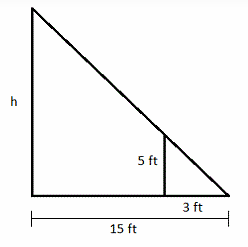$$\frac{3}{15}$$ = $$\frac{5}{h}$$
15 × 3 = 3h
75 = 3h
25 = h
h = 25

Question 10.
Justify Reasoning Are two equilateral triangles always similar? Explain.
yes; two equilateral triangles are always similar

Each angle of an equilateral triangle is 60°
Since both triangles are equilateral then they are similar

yes (see explanation)

Question 11.
Critique Reasoning Ryan calculated the missing measure in the diagram shown. What was his mistake?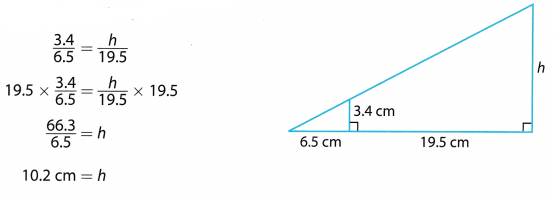In the first line, Ryan did not take the sum of 65 and 19.5 to get the denominator on the right

The denominator on the right should be 26 instead of 19.5

correct value for h
$$\frac{3.4}{6.5}$$ = $$\frac{h}{26}$$
26 × $$\frac{3.4}{6.5}$$ = $$\frac{h}{26}$$ × 26
$$\frac{88.4}{6.5}$$ = h
13.6 cm = h

error: 19.5 should be 26
h = 13.6
see explanation

Texas Go Math Grade 8 Lesson 7.3 H.O.T. Focus On Higher Order Thinking Answer Key

Question 12.
Communicate Mathematical Ideas For a pair of triangular earrings, how can you tell if they are similar? How can you tell if they are congruent?
The earrings are similar if two angle measures of one are equal to two angle measures of the other

The earrings are congruent if they are similar and if the side lengths of one are equal to the side lengths of the other.

similar → 2∠’S = 2∠’S
congruent → similar and side lengths = side lengths
see explanation

Question 13.
Critical Thinking When does it make sense to use similar triangles to measure the height and length of objects in real life?